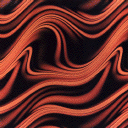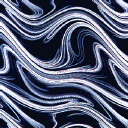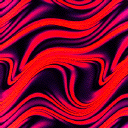# Pattern Formation

### Chaotic Advection of Passive Scalar

The distribution of dye in a fluid undergoing chaotic motion evolves in such a way that the gradient of the dye density increases with time and the region where the dye density gradient is largest becomes more and more striated into thinner and thinner ribbon-like regions, eventually concentrating on a fractal set. These pictures show the early stage of this process.
```(175K)(108K)(105K)
```
Reference: F. Varosi, T.M. Antonsen and E. Ott, "The spectrum of fractal dimensions of passive scalar gradients in chaotic fluid flows", Phys. Fluids A, vol. 3, p.1017 (1991)

### Two Dimensional Turbulence

Description: This picture shows the vorticity field in two dimensional turbulence with a drag force proportional to velocity added to the right hand side of the Navier-Stokes momentum equation. We find that the energy wavenumber spectum in the presence of drag scales like k-(3+n), n > 0, in contrast to the classical k-3 behavior without drag.
[K. Nam, et al., Phys. Rev. Lett., 84, 5134(2000)] (PDF) Description: This picture shows the blow-out solutions in two dimensional turbulence of Ginzburg-Landau Equation with a parameteric force. We find that the probability density function of the amplitude has a exponential tails. Description: The phase field resulting from a simulation of the complex Ginzburg-Landau equation. Several frames from a 100 x 100 domain with 512x512 grid points. See Target waves in the complex Ginzburg-Landau equation, Phys. Rev. E 62, 7627-7631 (2000) Description: A false color image of scalar that is passively advected by a chaotic fluid flow. The underlying flow has a spatial period that is one tenth that of the scalar. This gives rise to the small scale structure that is nearly periodic and modulated at the largest scale.